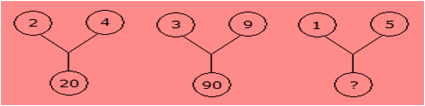# Humming Bird - HMO PDF Sample Papers for Class 8

Class 8 sample paper & practice questions for Humming Bird Mathematics Olympiad (HMO) level 1 are given below. Syllabus for level 1 is also mentioned for these exams. You can refer these sample paper & quiz for preparing for the exam.#### Resources:

##### Sample Questions from Olympiad Success:
 Q.1 Q.2 Q.3 Q.4 Q.5 Q.6 Q.7 Q.8 Q.9 Q.10
 Q.1 In equation 9x - 12k = 0. For x = 4, value of k is: a) 6 b) 5 c) 3 d) 8
 Q.2 Which of the following is not the property of a rectangle? a) a rectangle is a parallelogram in which one of  the angles is a right angle b) diagonals of a rectangle are equal c) opposite sides of a rectangle are equal d) diagonals of a rectangle bisect each other at right angle
 Q.3 Find the missing character from among the given alternatives: cx, fu, ir, ?, ol, ri a) lo b) mn c) no d) op
 Q.4 A car needs 54 litre of diesel for covering a distance of 297 km. The diesel required by the car to cover a distance of 550 km is: a) 100 litres b) 50 litres c) 25.16 litres d) 25 litres
 Q.5 Find the missing character from among the given alternatives:a) 75 b) 20 c) 25 d) 26
 Q.6 A four-digit number aabb is divisible by 55. Then possible value(s) of b is/are: a) 0 and 2 b) 2 and 5 c) 0 and 5 d) Can't be determined
 Q.7 The length of the arc of a sector of a circle is 17 cm and the area of the sector is 136 cm2. Calculate the radius of the circle. a) 16 cm b) 15 cm c) 14 cm d) 13 cm
 Q.8 Which is the smallest square number that is divisible by each of the number 4, 9 and10? a) 900 b) 810 c) 800 d) 920
 Q.9 How many diagonals have a regular hexagon? a) 3 b) 6 c) 9 d) 12
 Q.10 The ascending order of a = 0.025, b = 0.0035, c = 0.134 and d = 0.287 is: a) a, b, c, d b) b, a, c, d c) b, c, d, a d) c, a, b, dSample PDF of Humming Bird - Humming Bird Mathematics Olympiad (HMO) PDF Sample Papers for Class 8:

 Q.1 )c Q.2 )d Q.3 )a Q.4 )a Q.5 )d Q.6 )c Q.7 )a Q.8 )a Q.9 )c Q.10 )b

Q.1 : c | Q.2 : d | Q.3 : a | Q.4 : a | Q.5 : d | Q.6 : c | Q.7 : a | Q.8 : a | Q.9 : c | Q.10 : b